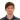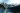Back

TechnologyMay 10, 2017

# Simulating Server Errors Using a Poisson ProcessKevin WheelerWhen a microservice generates errors, someone needs to look into it. But determining how many errors is worth notifying on-call staff is an overlooked decision. Instead of just guessing values, there’s a scientific way to determine the optimal threshold.

### THE CHALLENGE

A previous client sent automated alerts to on-call staff when a microservice in their system surpassed a threshold of too many errors of a certain type (e.g., too many HTTP 500 Responses as measured by the exponential moving average.) However, the same default thresholds were applied to all the microservices. Some microservices needed more tolerance for failures due to various external factors (e.g., if there is a dependency on other systems outside of the client’s control). The challenge was finding what the threshold should be using a simple model rather than guessing values in production.

A simple simulation using basic probability distributions was the solution. Knowing how these simulations affected the automated alerts allowed the ability to set thresholds for the actual microservice in a scientific manner.

### THE MODEL

A model for a request to a microservice is the exponential distribution. This is the distribution that simulates the time between occurrences of successive events. For example, the exponential distribution could be used to simulate the time between goals in a World Cup soccer match (as noted in the Wikipedia article). The input is the variable lambda, which represents the rate of arrivals; in the soccer match lambda might be one goal per 30 minutes. The expected average number of requests per minute would be the appropriate value for the microservice example.

There are libraries that allow for simulation of these values, but a simple way to generate a list of times between events is as follows (using the inverse transform method):

`double ``lambda = 60; ``//i.e. 60 events/minute```` List<Double> timesBetweenEvents = ````new ````ArrayList<>(120); ````for ``(``int ````i=0; i<120; i++) { ``````//log in this case is the natural log (ln) ````double ``timeBetweenEvent = -(1/lambda)*Math.``log``(Math.``random````()); timesBetweenEvents.add(timeBetweenEvent); } ````return ``timesBetweenEvents;`

In this example, lambda is set to 60 requests per minute. There are 120 events being simulated (which is going to be approximately two minutes of events.)

After generating the times between events, the question was how to define if that mocked event would be a failure (i.e., if it should even be tallied up for measure against the threshold). Based on the assumption that the failures are evenly distributed in normal business scenarios, the uniform distribution was used. The right failure ratio is related to the external dependency’s down time. In this example, the failure rate is 2%. The code is below:

`double ````cutoff = .02; ````if ``(Math.``random````() > cutoff) { ````return false````; } ``````else ``````{ ````return true````; }```

Math.random() will return a number between 0 and 1. If the number is below .02 then that is considered a failure. To simplify this, if the cutoff were set to 0.5 this would be the same as flipping a coin to decide if the event is a failure. With it set to .02 it is just a biased coin flip.

### THE RESULT

With these two probability distributions, the simulation can be run repeatedly and the thresholds manipulated. This allows a more repeatable, scientific approach to defining on-call alerts. This also allows the numbers to be tweaked to test events outside of normal business scenarios or load testing before they occur. The underlying math would remain the same, only the lambda and failure ratio would change. As a next step, for greater accuracy, the model can be tested against actual production data to ensure fit.